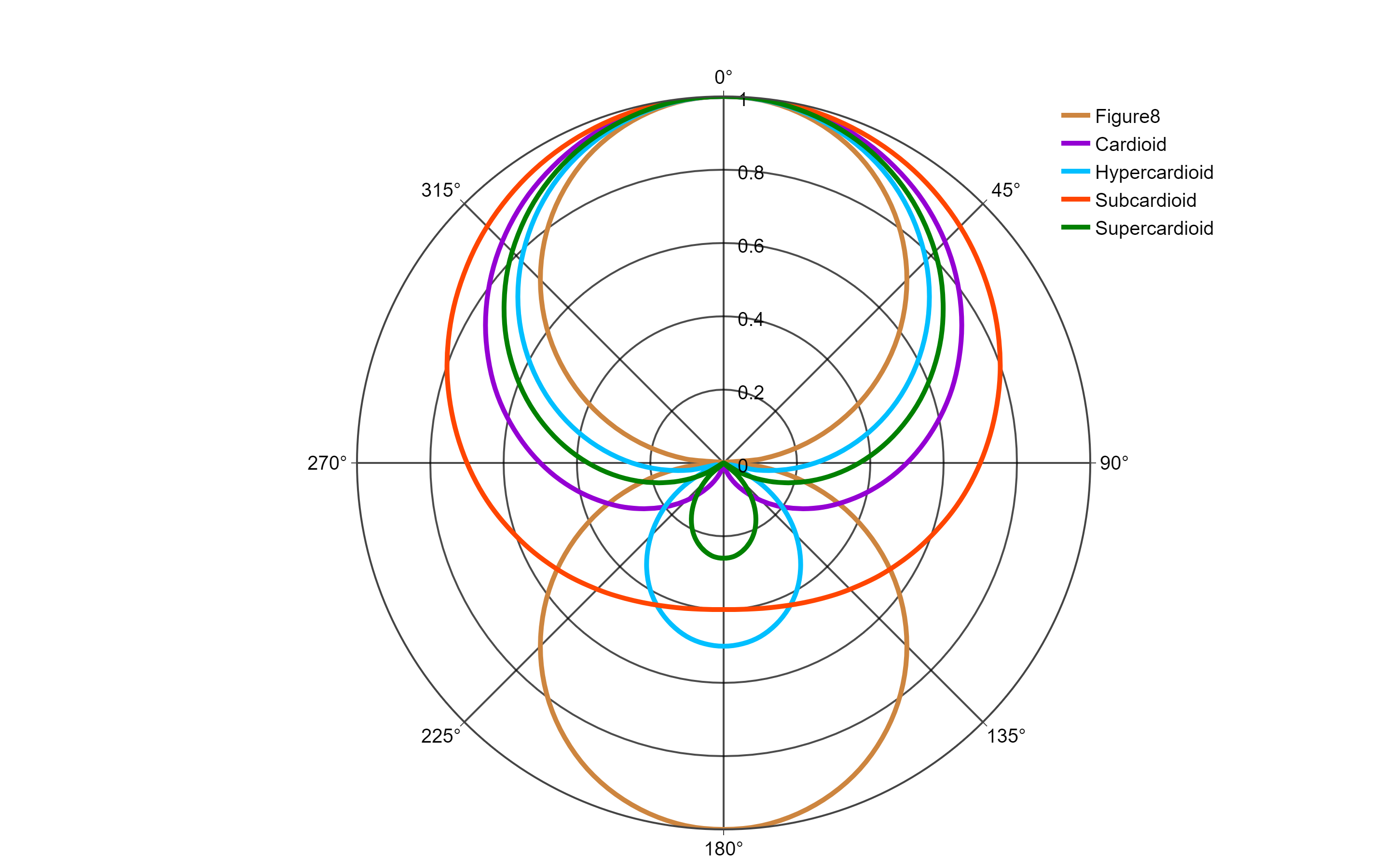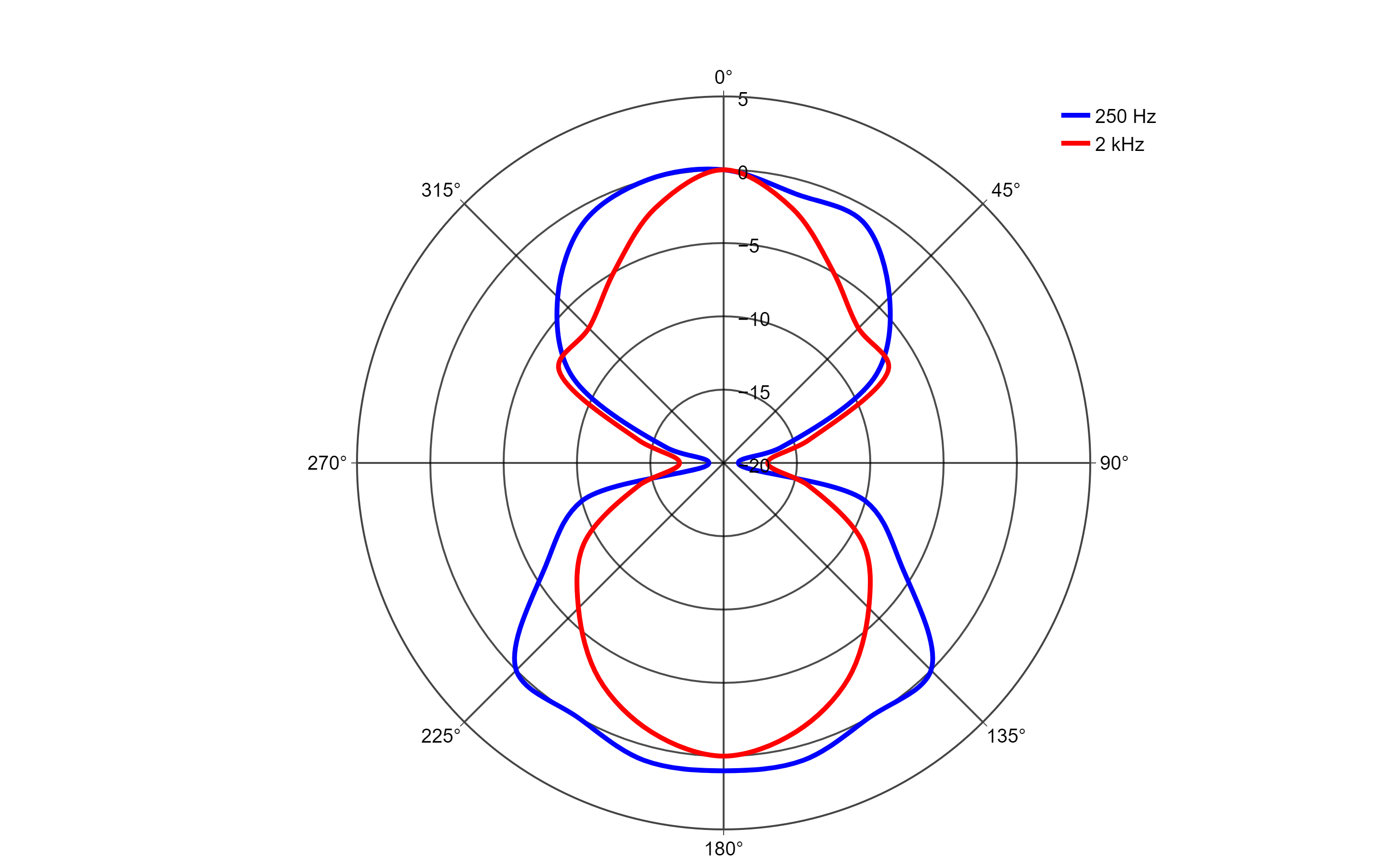# Microphone Polar PlotsIn this example we will plot some idealised polar patterns followed by some measured polar data.

Data is stored in a csv file where the 1st column contains a list of angles and subsequent columns contain sound pressure levels for each trace. In the idealised example the patterns are constructed from numbers ranging from 0 to 1. In the measured example the sound pressure levels have been normalised to show deviations in level at each angle from the on axis position.

# Idealised Polar Patterns

Read in the example csv file using:

```myFile <- file.choose()

Now we can create a ‘scatterpolar’ plot and add each trace to it. This example contains patterns for:

• Cardioid
• Hypercardioid
• Subcardioid
• Supercardioid
• Figure of eight

Under line use:

` shape = "spline",`

To smooth each trace.

Under layout > polar you will need to edit parameters under radialaxis and angularaxis to change the number of ticks, their colour, thickness, and orientation. To change the axis scale use range under radialaxis.# Measured Polar Patterns

This example modifies the previous example script to plot measured polar data that has been normalised.

The scale has been modified to range between +5 dB and -20 dB.